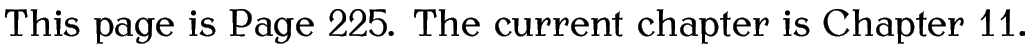# 11. Counters

As we have seen, LaTeX automatically generates numbers for chapters, sections, equations etc. These numbers are stored in counters. The names of these counters are usually the same as the name of the object with which it is associated but without any backslash. For example, the \chapter command has an associated counter called chapter, the \footnote command has an associated counter called footnote, the equation environment has an associated counter called equation, the figure environment has an associated counter called figure and the table environment has an associated counter called table. There is also a counter called page that keeps track of the current page number.

The value of a counter can be displayed using the command

\the<counter>

where <counter> is the name of the associated counter. Note that <counter> does not go in curly braces and adjoins \the (for example, \thepage[Page number is wrong at start of page], \thesection or \thechapter). In fact, we have already encountered \thefigure in §7.4. Sub-Floats.

Example:

This page is Page~\thepage.
The current chapter is Chapter~\thechapter.New counters can be created using the command:

\newcounter{<counter-name>}[<outer-counter>]

The mandatory argument <counter-name> is the name of your new counter (no backslash in the name). For example, let's define a counter called exercise to keep track of each exercise. (Recall the exercise example from §10. Defining Environments.)

\newcounter{exercise}

We can now display the value of the counter using the command \theexercise. At the moment the counter has the value zero, the value can be changed using one of the following commands:

\stepcounter{<counter>}
Increments <counter> by 1

\refstepcounter{<counter>}
As above, but allows you to cross-reference the counter using \label and \ref

\setcounter{<counter>}{<num>}
Sets the counter to <num>

\addtocounter{<counter>}{<num>}

A couple of the commands above take a number <num> as one of the arguments. If you want to use another counter for this argument, you need to use

\value{<counter>}

For example, if you want to set our new exercise counter to the same value as the page counter, you would do

\setcounter{exercise}{\value{page}}

Let's go back to the exercise environment you created in Exercise 25. The exercises really ought to have an associated number, and this number should be incremented each time we use the exercise environment. So let's modify our code to do this. Modifications are illustrated in bold like this:

\newcounter{exercise}

\newenvironment{exercise}% environment name
{% begin code
\par\vspace{\baselineskip}\noindent
\refstepcounter{exercise}%
\textbf{Exercise \theexercise\␣(#1)}%
\begin{itshape}%
\par\vspace{\baselineskip}%
\noindent\ignorespaces
}
%
{% end code
\end{itshape}%
\par\vspace{\baselineskip}%
\noindent\ignorespacesafterend
}

Note that the counter needs to be incremented before it is used. I've also added an extra \vspace at the end of the environment and a paragraph break. Since we've used \refstepcounter instead of \stepcounter we can cross-reference our exercise environment:

Exercise~\ref{ex:simple} is a simple exercise.

\begin{exercise}{Simple Exercise}
\label{ex:simple}%
This is a simple exercise.
\end{exercise}

This produces the following output:The counter representation can be changed by redefining \theexercise[Redefining counters' \the-commands] using the \renewcommand command described in §8.2. Redefining Commands. The following commands can be used to display the counter:

\arabic{<counter>}
Arabic numeral (1, 2, 3, ...)

\Roman{<counter>}
Upper case Roman numeral (I, II, III, ...)

\roman{<counter>}
Lower case Roman numeral (i, ii, iii, ...)

\alph{<counter>}
Lower case letter (a, b, c, ..., z)

\Alph{<counter>}
Upper case letter (A, B, C, ..., Z)

\fnsymbol{<counter>}
A footnote symbol ()

Example:

To make the chapter numbers appear as upper case Roman numerals you would do:

You may have noticed that \newcounter has an optional argument <outer-counter>. This is for use if you require the new counter to be reset every time <outer-counter> is incremented[Master and slave counters]. For example, the section numbers in the scrbook class are dependent on the chapter numbers. Each time a new chapter is started, the section numbers are reset. Suppose we want our exercise counter to be dependent on the chapter counter, we would do

\newcounter{exercise}[chapter]

Note that if you make a counter dependent on another counter like this, the default action of \the<counter> remains the same, so \theexercise won't print the chapter number. To make the chapter number appear as well, we need to redefine \theexercise (recall §8.2. Redefining Commands):

\renewcommand{\theexercise}{\thechapter.\arabic{exercise}}

Notice the use of \thechapter instead of, say, \arabic{chapter}. This way we don't need to keep track of the chapter counter format.

Example (Footnote Markers):

The footnote counter is reset at the start of each chapter but by default the chapter number isn't displayed in \thefootnote. In the PDF version of this document \thefootnote was redefined so that it displays the chapter number:

Exercise 26: Using Counters

Modify the document from Exercise 25 so that the exercise environment has a counter. Make the counter dependent on the chapter. You can download or view an example.

This book is also available as A4 PDF or 12.8cm x 9.6cm PDF or paperback (ISBN 978-1-909440-00-5).

© 2012 Dickimaw Books. "Dickimaw", "Dickimaw Books" and the Dickimaw parrot logo are trademarks. The Dickimaw parrot was painted by Magdalene Pritchett.